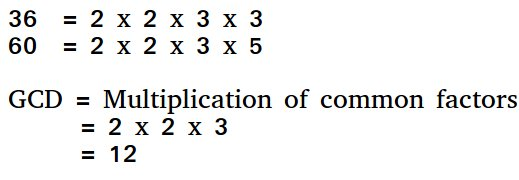GeeksforGeeks App
Open AppBrowser
Continue

# Program to find HCF iteratively

HCF (Highest Common Factor) or GCD (Greatest Common Divisor) of two numbers is the largest number that divides both of them.For example, GCD of 20 and 28 is 4, and GCD of 98 and 56 is 14.

We have discussed the recursive solution in the below post. Recursive program to find GCD or HCF of two numbers
Below is the iterative implementation of Euclid’s algorithm

## C++

 `// C++ program to find HCF of two``// numbers iteratively.``#include ``using` `namespace` `std;` `int` `hcf(``int` `a, ``int` `b)``{``    ``if` `(a == 0)``        ``return` `b;``    ``else` `if` `(b == 0)``        ``return` `a;``    ``while` `(a != b) {``        ``if` `(a > b)``            ``a = a - b;``        ``else``            ``b = b - a;``    ``}``    ``return` `a;``}` `// Driver code``int` `main()``{``    ``int` `a = 60, b = 96;``    ``cout << hcf(a, b) << endl;``    ``return` `0;``}` `// This code is contributed by shubhamsingh10`

## C

 `// C program to find HCF of two``// numbers iteratively.``#include ` `int` `hcf(``int` `a, ``int` `b)``{``    ``if` `(a == 0)``        ``return` `b;``    ``else` `if` `(b == 0)``        ``return` `a;``    ``while` `(a != b) {``        ``if` `(a > b)``            ``a = a - b;``        ``else``            ``b = b - a;``    ``}``    ``return` `a;``}``int` `main()``{``    ``int` `a = 60, b = 96;``    ``printf``(``"%d\n"``, hcf(a, b));``    ``return` `0;``}`

## Java

 `// JAVA Code for Program to find``// HCF iteratively``import` `java.util.*;` `class` `GFG {` `    ``static` `int` `hcf(``int` `a, ``int` `b)``    ``{``        ``if` `(a == ``0``)``            ``return` `b;``        ``else` `if` `(b == ``0``)``            ``return` `a;``        ``while` `(a != b) {``            ``if` `(a > b)``                ``a = a - b;``            ``else``                ``b = b - a;``        ``}``        ``return` `a;``    ``}` `    ``/* Driver program */``    ``public` `static` `void` `main(String[] args)``    ``{``        ``int` `a = ``60``, b = ``96``;``        ``System.out.println(hcf(a, b));``    ``}``}` `// This code is contributed by Arnav Kr. Mandal.`

## Python3

 `/``/` `Python program to find HCF of two``/``/` `numbers iteratively.`  `def` `hcf(a, b):``    ``if``(a ``=``=` `0``):``      ``return` `b``    ``else` `if``(b ``=``=` `0``):``      ``return` `a``    ``while` `(a !``=` `b):``        ``if` `(a > b):``            ``a ``=` `a ``-` `b``        ``else``:``            ``b ``=` `b ``-` `a``    ``return` `a`  `a ``=` `60``b ``=` `96``print``(hcf(a, b))`

## C#

 `// C# Code for Program to find``// HCF iteratively``using` `System;` `class` `GFG {` `    ``static` `int` `hcf(``int` `a, ``int` `b)``    ``{``        ``if` `(a == 0)``            ``return` `b;``        ``else` `if` `(b == 0)``            ``return` `a;``        ``while` `(a != b) {``            ``if` `(a > b)``                ``a = a - b;``            ``else``                ``b = b - a;``        ``}``        ``return` `a;``    ``}` `    ``// Driver program``    ``public` `static` `void` `Main()``    ``{``        ``int` `a = 60, b = 96;``        ``Console.WriteLine(hcf(a, b));``    ``}``}` `// This code is contributed by vt_m.`

## PHP

 ` ``\$b``)    ``            ``\$a` `= ``\$a` `- ``\$b``;    ``        ``else``            ``\$b` `= ``\$b` `- ``\$a``;    ``    ``}``    ` `    ``return` `\$a``;``}` `// Driver code``\$a` `= 60; ``\$b` `= 96;``echo` `hcf(``\$a``, ``\$b``), ``"\n"``;``    ` `// This code is contributed by ajit``?>`

## Javascript

 ``

Output:

`12`

Time Complexity: O(max(a, b))

Auxiliary Space: O(1)

My Personal Notes arrow_drop_up